## Ages for SBI PO Set – 1

1. The ratio between the ages of a father and a son at present is 5:2, respectively. Four years, hence the ratio between the ages of the son and his mother will be 1:2 respectively. What is the ratio between the present ages of the father and the mother respectively?

(a) 3:4

(b) 5:4

(c) 4:3

(d) Cannot be determined

(d) Cannot be determined

Explanation:

Suppose the ages of father and son are 5x and 2x years.

After four years the age of son = 2x + 4

After four years the age of mother = 4x + 8

So the present age of mother = 4x + 4

Ratio of the age of father and mother = 5x : (4x + 4)

Data are insufficient, so cannot be determined.

2. Radha’s present age is three years less than twice her age 12 years ago. Also the respective ratio between Raj’s present age and Radha’s present age in 4:9. What will be Raj’s age after 5 years?

(a) 12 years

(b) 7 years

(c) 21 years

(d) None of these

(d) None of these

Explanation:

Present age of Radha = x years

According to the question,

x + 3 = 2(x – 12)

x + 3 = 2x – 24

x = 27

Present age of Raj : Present age of Radha = 4 : 9

Therefore, Present age of Raj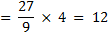After 5 years age of Raj = 12 + 5 = 17 years

3. The respective ratio of the present ages of Swati and Trupti is 4:5. Six years hence the respective ratio of their ages will be 6:7. What is the difference between their ages?

(a) 2 years

(b) 3 years

(c) 4 years

(d) Cannot be determined

(b) 3 years

Explanation:

(S+6)/(T+6) = 6/7

7S + 42 = 6T + 36

7S – 6T = 36 – 42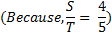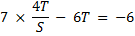(28T-30T)/5 = -6

-2T = -30

Therefore, T = 15 then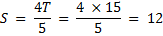Difference = 15 – 12 = 3 years

4. Four years ago Shayam’s age was three-fourths times that of Ram. Four years hence, Shyam’s age will be five-sixths times that of Ram. What is the present age of Shyam?

(a) 15 years

(b) 20 years

(c) 16 years

(d) 24 years

(c) 16 years

Explanation:

Age before 4 years

Shyam : Ram = 3 : 4

After 4 years, age is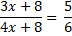20x + 40 = 18x + 48

20x – 18x = 48 – 40

2x = 8

X = 4

5. The ratio of the present ages of Anju and Sandhya is 13:17, respectively. Four years ago the respective ratio of their ages was 11:15. What will be the respective ratio of their ages six years hence?

(a) 3:4

(b) 7:8

(c) 5:4

(d) None of these

(d) None of these

Explanation: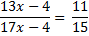195x – 60 = 187x – 44

195x – 187x = 60 – 44

8x = 16

X = 2

Ratio of their ages after 6 years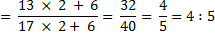6. The ages of Sulekha and Arunima are in the ratio of 9:8 respectively. After 5 years the ratio of their ages will be 10:9. What is the difference in years between their ages?

(a) 4 years

(b) 5 years

(c) 6 years

(d) 7 years

(b) 5 years

Explanation:

Suppose the age of Sulekha and Arunima is 9x and 8x.

Therefore,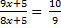81x + 45 = 80x + 50

81x – 80x = 50 – 45

X = 5

Therefore, difference = 9 × 5 – 8 × 5

= 45 – 40 = 5 years

7. The present ages of Amit and his father are in the ratio of 2:5, respectively. Four years hence the ratio of their ages will become 5:11, respectively. What was the father’s age five years ago?

(a) 40 years

(b) 45 years

(c) 30 years

(d) 35 years

(d) 35 years

Explanation:

Suppose the present age of Amit and his father is 2x and 5x years respectively.

After 4 years the ratio of their ages,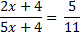25x + 20 = 22x + 44

25x – 22x = 44 – 20

3x = 24

X = 8

Age of his father before 5 years

= 5x – 5

= 5(8) – 5

= 40 – 5

= 35 years

8. The respective ratio between the present age of Manisha and Deepali is 5:x. Manisha is 9 years younger than Parineeta. Parineeta’s age after 9 years will be 33 years. The difference between Deepali’s and Manisha’s age is same as the present age of Parineeta. What will come in place of x?

(a) 23

(b) 39

(c) 15

(d) None of these

(d) None of these

Explanation:

Given parineeta’s age after 9 years = 33 years

Therefore, Parineeta’s present age = 33 – 9 = 24 years

Therefore, Manisha’s present age = 24 – 9 = 25 years

Therefore, Deepali’s present age = 15 + 24 = 39 years

Hence, ratio between Manisha and Deepali

= 15 : 39 = 5 : 13

X = 13

9. Michelle got married 9 years ago. Today her age is 1(1/3) times her age at the time of marriage. At present her daughter’s age is one-sixth of her age. What was her daughter’s age two years ago?

(a) 6 years

(b) 7 years

(c) 3 years

(d) None of these

(d) None of these

Explanation:

Suppose at the time of marriage, the age of Michelle was x years.

x + 9 = (4/9)x

x = 27 years

Therefore, present age of Michelle

= 27 + 9 = 36 years

Here daughter’s age two years ago

= 36/6 – 2 = 6 – 2 = 4 years

10. The respective ratio between the present ages of Ram and Rakesh is 6:11. Four years ago, the ratio of their ages was 1:2, respectively. What will be Rakesh’s age after five years?

(a) 45 years

(b) 29 years

(c) 49 years

(d) Cannot be determined

(c) 49 years

Explanation:

Let the age of Ram = x and Rakesh = y then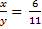Therefore,

x = 6y/11

According to the question,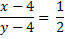2x – 8 = y – 4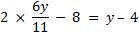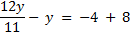y/11 = 4

Y = 44 years

Therefore, age of Rakesh after 5 years

= 44 + 5 = 49 years.